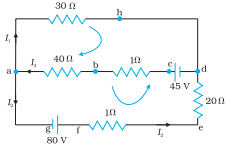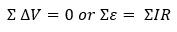Electric circuits generally consist of a number of resistors and cells interconnected sometimes in a complicated way. The formulae we have derived earlier for series and parallel combinations of resistors are not always sufficient to determine all the currents and potential differences in the circuit. Before understanding these laws, we first define a few terms.

1. Electric network: The term electric network is used for a complicated system of electrical conductors.
2. Junction: Any point in an electric circuit where two or more conductors are joined together is a junction.
3. Loop or Mesh: Any closed conducting path in an electric network is called a loop or mesh.
4. Branch: A branch is any part of the network that lies between two junction.

### Kirchhoff’s first law or junction rule:

In an electric circuit, the algebraic sum of currents at any junction is zero. Or, the sum of currents entering a junction is equal to the sum of currents leaving that junction.

Mathematically, this law may be expressed as#### Sign convention for applying junction rule:

1. The current flowing towards the junction are taken as positive.
2. The currents flowing away from the junction are taken as negative.Fig. At junction a the current leaving is I1 + I2 and current entering is I3. The junction rule says I3 = I1 + I2. At point h current entering is I1. There is only one current leaving h and by junction rule that will also be I1. For the loops ‘ahdcba’ and ‘ahdefga’, the loop rules give –30I1 – 41 I3 + 45 = 0 and –30I1 + 21 I2 – 80 = 0.

### Kirchhoff’s second law or loop rule:

Around any closed loop of a network, the algebraic sum of charges in potential must be zero. Or, the algebraic sum of the emfs in any loops of a circuit is equal to the sum of the products of currents and resistance in it.

Mathematically, the loop rule may be expressed as#### Sign convention for applying loop rule:

1. We can take any direction (clockwise or anticlockwise) as the direction of traversal.
2. The emf of cell is taken as positive if the direction of traversal is from its negative to the positive terminal (through the electrolyte).